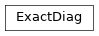# ExactDiag¶

Inheritance DiagramMethods

 `ExactDiag.__init__`(model[, charge_sector, ...]) Calculate self.full_H from self.mpo. Calculate self.full_H from self.mpo. Return `U(dt) := exp(-i H dt)`. `ExactDiag.from_H_mpo`(H_MPO, *args, **kwargs) Wrapper taking directly an MPO instead of a Model. `ExactDiag.full_diagonalization`(*args, **kwargs) Full diagonalization to obtain all eigenvalues and eigenvectors. `ExactDiag.full_to_mps`(psi[, canonical_form]) Convert a full state (with a single leg) to an MPS. `ExactDiag.groundstate`([charge_sector]) Pick the ground state energy and ground state from `self.V`. Allow to use self as LinearOperator for lanczos. Contract an MPS along the virtual bonds and combine its legs. `ExactDiag.sparse_diag`(k, *args, **kwargs) Call `speigs()`.
class tenpy.algorithms.exact_diag.ExactDiag(model, charge_sector=None, sparse=False, max_size=2000000.0)[source]

Bases: `object`

(Full) exact diagonalization of the Hamiltonian.

Parameters
• model (`MPOmodel` | `CouplingModel`) – The model which is to be diagonalized.

• charge_sector (`None` | charges) – If not `None`, project onto the given charge sector.

• sparse (bool) – If `True`, don’t sort/bunch the LegPipe used to combine the physical legs. This results in array blocks with just one entry, requires much more charge data, and is not what np_conserved was designed for, so it’s not recommended.

• max_size (int) – The build_H_* functions will do nothing (but emit a warning) if the total size of the Hamiltonian would be larger than this.

model

The model which is to be diagonalized.

Type

`MPOmodel` | `CouplingModel`

chinfo

The nature of the charge (which is the same for all sites).

Type

`ChargeInfo`

charge_sector

If not `None`, we project onto the given charge sector.

Type

`None` | charges

max_size

The `build_H_*` functions will do nothing (but emit a warning) if the total size of the Hamiltonian would be larger than this.

Type

int

full_H

The full Hamiltonian to be diagonalized with legs `'(p0.p1....)', '(p0*,p1*...)'` (in that order). `None` if the `build_H_*` functions haven’t been called yet, or if max_size would have been exceeded.

Type

`Array` | `None`

E

1D array of eigenvalues.

Type

ndarray | `None`

V

Eigenvectors. First leg ‘ps’ are physical legs, the second leg `'ps*'` corresponds to the eigenvalues.

Type

`Array` | `None`

_sites

The sites in the given order.

Type

list of `Site`

_labels_p

The labels use for the physical legs; just `['p0', 'p1', ...., 'p{L-1}']`.

Type

list or str

_labels_pconj

Just each of _labels_p with an `*`.

Type

list or str

_pipe

The pipe from the single physical legs to the full combined leg.

Type

`LegPipe`

_pipe_conj

Just `_pipe.conj()`.

Type

`LegPipe`

Bool mask, which of the indices of the pipe are in the desired charge_sector.

Type

1D bool ndarray | `None`

classmethod from_H_mpo(H_MPO, *args, **kwargs)[source]

Wrapper taking directly an MPO instead of a Model.

Parameters
• H_MPO (`MPO`) – The MPO representing the Hamiltonian.

• *args – Further keyword arguments as for the `__init__` of the class.

• **kwargs – Further keyword arguments as for the `__init__` of the class.

build_full_H_from_mpo()[source]

Calculate self.full_H from self.mpo.

build_full_H_from_bonds()[source]

Calculate self.full_H from self.mpo.

full_diagonalization(*args, **kwargs)[source]

Full diagonalization to obtain all eigenvalues and eigenvectors.

Arguments are given to `eigh`.

groundstate(charge_sector=None)[source]

Pick the ground state energy and ground state from `self.V`.

Parameters

charge_sector (None | 1D ndarray) – By default (`None`), consider all charge sectors. Alternatively, give the qtotal which the returned state should have.

Returns

• E0 (float) – Ground state energy (possibly in the given sector).

• psi0 (`Array`) – Ground state (possibly in the given sector).

exp_H(dt)[source]

Return `U(dt) := exp(-i H dt)`.

mps_to_full(mps)[source]

Contract an MPS along the virtual bonds and combine its legs.

Parameters

mps (`MPS`) – The MPS to be contracted.

Returns

psi – The MPS contracted along the virtual bonds.

Return type

`Array`

full_to_mps(psi, canonical_form='B')[source]

Convert a full state (with a single leg) to an MPS.

Parameters
Returns

mps – An normalized MPS representation in canonical form.

Return type

`MPS`

matvec(psi)[source]

Allow to use self as LinearOperator for lanczos.

Just applies full_H to (the first axis of) the given psi.

sparse_diag(k, *args, **kwargs)[source]

Call `speigs()`.